# How to calculate the percentage of year or month passed in Excel?

Assume you have a set of dates on a spreadsheet, and you want to calculate the percentage of the year or month that has already passed. How would you go about doing this? According to the date that was provided. How might one go about finding a solution to this task using Excel?

In this tutorial, we will show how to use the DAY function, as well as the EOMONTH function, and YEARFRAC function. Additionally, we will calculate something using a general mathematical equation.

## Calculating the Percentage of Month Passed in Excel using Simple Formulas

The following set of formulas can be of use to you in calculating the percentage of the year that has passed since the given date.

Let’s understand step by step with an example.

### Step 1

Let’s assume, we have a sample data. Refer to the below screenshot.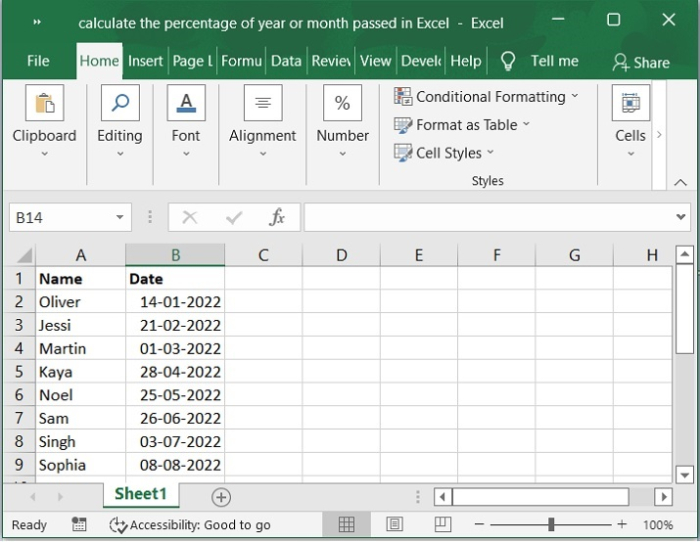Combining the DAY function with the EOMONTH function is the simplest way to get the percentage of the month that has passed since the beginning of the month. Let's say we have a list of dates similar to the information below, and we want to determine the percentage of each month that has already passed.

### Step 2

Now, enter the following formula into a blank cell where you wish to put the result and press enter key. Please refer to the below screenshot for the same.

=DAY(B2)/DAY(EOMONTH(B2,0))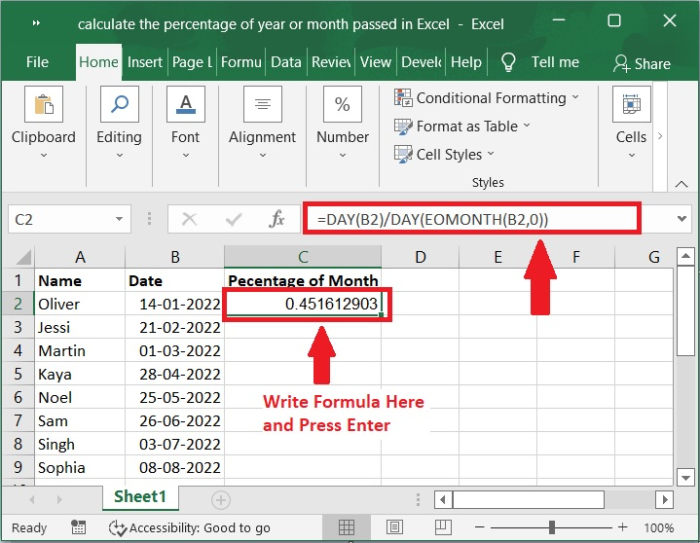### Step 3

After that, slide the fill handle down to fill the rest of the cells, and you will obtain some decimal numbers, as seen in the screenshot below.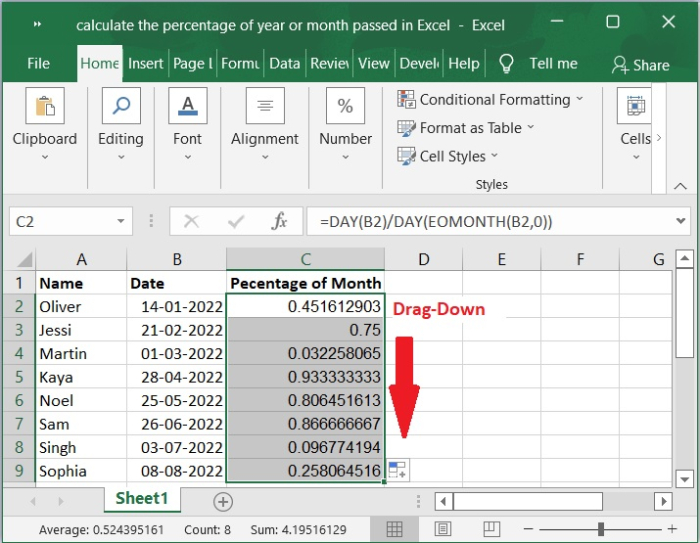### Step 4

To get the values in percentage format, select the data and Click Number group choose % (Percentage) symbol so that cells will get formatted. Please check out the screenshot below.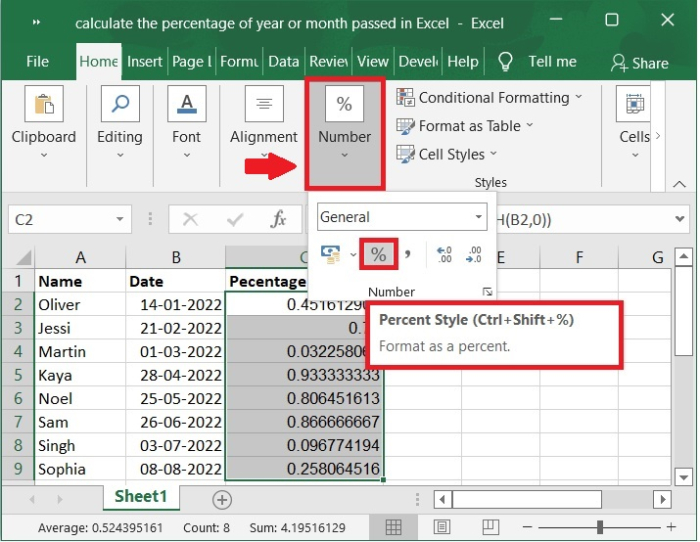### Step 5

Once you click on the percentage (%) symbol. You will get the values in % format as shown in the below screenshot for the same.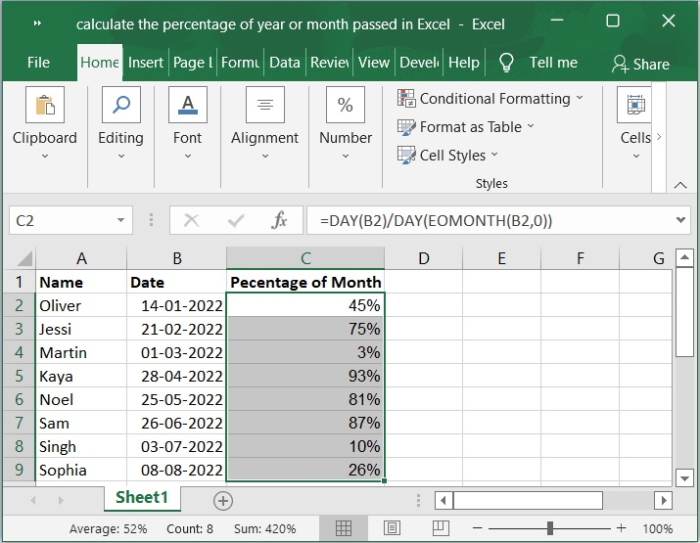## Calculating the Percentage of Year Passed in Excel Using a Function

We'll now going to look at another helpful function called the YEARFRAC function, which calculates. In this case, we have several dates from various months; therefore, we will determine the percentage of the month that has passed since that particular date by looking at how much of the year has passed since that date.

### Step 6

Now, enter the formula into a blank cell where you wish to put the result and press enter key. Please refer to the below screenshot for the same.

=YEARFRAC(DATE(YEAR(B2),1,1),B2)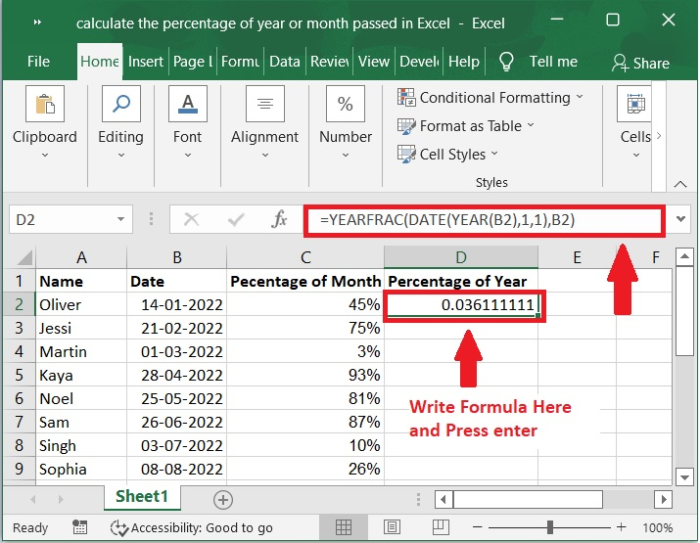### Step 7

After that, slide the fill handle down to fill the rest of the cells, and you will obtain some decimal numbers, as seen in the screenshot below.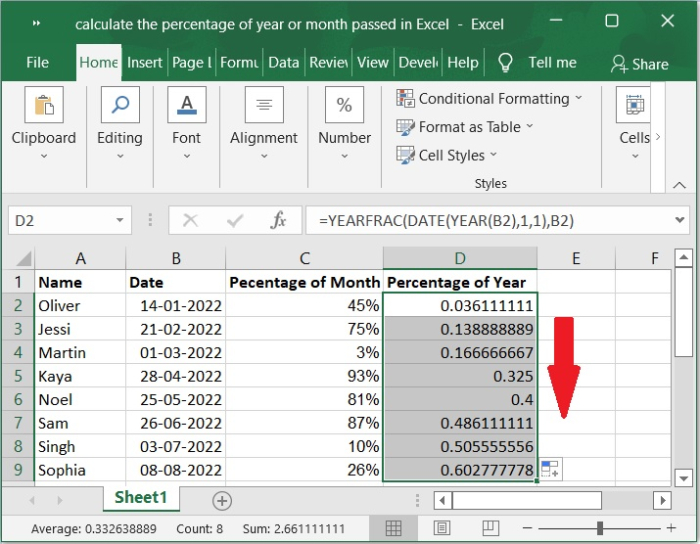### Step 8

To get the values in percentage format, select the data and Click Number group choose % (Percentage) symbol so that cells will get formatted. Once you click on the percentage (%) symbol. You will get the values in % format as shown in the below screenshot for the same.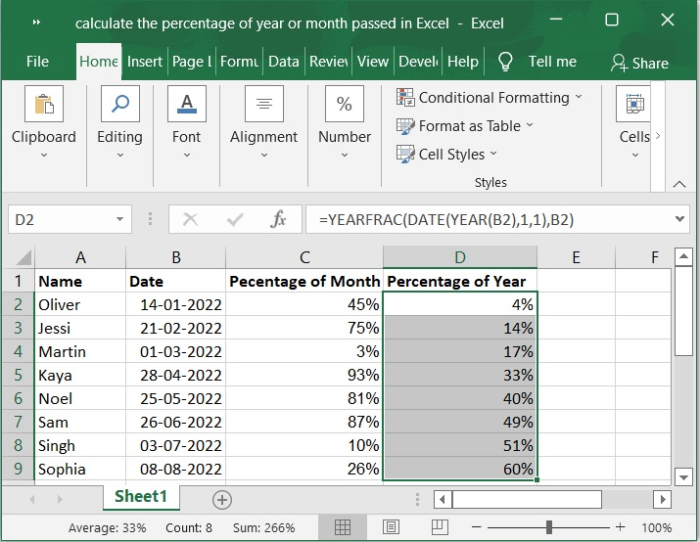## Conclusion

In this tutorial, we used a simple example to show how you can calculate the percentage of year or month passed in Excel by using simple formulas.

Updated on: 06-Feb-2023

512 Views